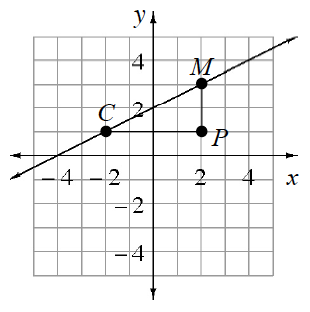### Home > INT2 > Chapter 3 > Lesson 3.1.3 > Problem3-31

3-31.

Examine the graph of line $\overleftrightarrow { C M }$at right.

1. Write the equation of $\overleftrightarrow { C M }$.

Use the slope triangle in the diagram to determine the slope of the line.

2. What are the $x$- and $y$-intercepts of the line?

3. Calculate the area and perimeter of $ΔCPM$.

Area $= 4$ units$^2$

4. Write an equation of the line through point $M$ that is perpendicular to $\overleftrightarrow { C M }$.

The slopes of perpendicular lines are negative reciprocals.